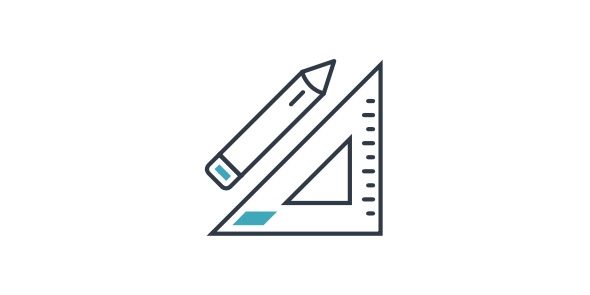# Ultimate Quiz On 3rd Grade Math

16 Questions | Total Attempts: 121Settings• 1.
What is 10 x 6?
• A.

16

• B.

106

• C.

601

• D.

60

• 2.
What is 11 x 5?
• A.

511

• B.

16

• C.

115

• D.

55

• 3.
What is 96 divided by 12?
• A.

8

• B.

84

• C.

108

• D.

9

• 4.
What is 12 x 12?
• A.

441

• B.

1212

• C.

24

• D.

144

• 5.
What is 500 + 30 + 7?
• A.

537

• B.

573

• C.

500307

• D.

735

• 6.
How many inches are in 2 linear feet?
• A.

48 Inches

• B.

18 Inches

• C.

24 Inches

• D.

12 Inches

• 7.
What number comes next in the sequence 200, 400, 600, 800?
• A.

800

• B.

1200

• C.

1000

• D.

600

• 8.
What is 7 x 11?
• A.

711

• B.

18

• C.

17

• D.

77

• 9.
What is 8 x 12?
• A.

96

• B.

69

• C.

128

• D.

20

• 10.
How would you write 3 1/3 as an improper fraction?
• A.

31/3

• B.

3-Oct

• C.

10-Mar

• D.

3-Jan

• 11.
What is 3 x 11?
• A.

14

• B.

311

• C.

33

• D.

31

• 12.
What is 72 divided by 6?
• A.

432

• B.

12

• C.

78

• D.

66

• 13.
What is 736 + 29?
• A.

766

• B.

765

• C.

756

• D.

755

• 14.
What is 7/9 + 1/9?
• A.

18-Aug

• B.

9-Aug

• C.

8-Sep

• D.

18/8

• 15.
What is 5 x 9?
• A.

59

• B.

95

• C.

45

• D.

14

• 16.
What is 16 divided by 2?
• A.

18

• B.

32

• C.

14

• D.

8

Related Topics